# Sritharan, R.

## The stubborn list partition problem ★★

Author(s): Cameron; Eschen; Hoang; Sritharan

Problem   Does there exist a polynomial time algorithm which takes as input a graphand for every vertex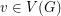a subset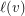of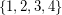, and decides if there exists a partition ofinto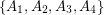so that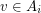only ifand so thatare independent,is a clique, and there are no edges betweenand?

Keywords: list partition; polynomial algorithm